# Circular Motion

Is this pawn accelerating?

1. Yes
2. No
3. Not so sureNow let's consider an object moving with the same speed along the circular path.

At any given point, the velocity vector is both tangent to the circle and has the same length at all positions.

The total time that it takes for this object to go around the circle once the period, $T$.

Thus, we can say the speed (the magnitude of the velocity) is

$$v =\frac{\textrm{distance traveled}}{\textrm{time it took}}= \frac{2\pi r}{T}$$

Find the speed of the moon as it orbits around the earth. The moon is on avarage 385,000 km from the earth. It take 27.3 days to complete one orbit.

`

### Uniform Circular Motion:

If we can say that the speed of an object undergoing circular motion is not changing, then we'll call that type of motion: Uniform Circular Motion.Some examples: planets going around the sun. (mostly circular although not quite)

A record player.

horses on the carousel (while the ride is not starting or stopping.)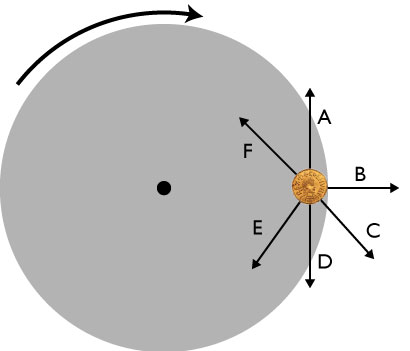A coin sits on a rotating turntable. The direction of rotation is shown by the curved arrow above the turntable. (You are looking down on the turntable.) At the instant shown in the figure, which arrow is closest to the direction of the coin’s velocity?Let's find $\Delta \mathbf{v}$:

$$\Delta \mathbf{v} = \mathbf{v} - \mathbf{v}_0$$

Graphical subtraction would look like this:

Which vector best represents $\mathbf{v} - \mathbf{v}_0$ ?Which vector best represents $\mathbf{v} - \mathbf{v}_0$ ?Now we can see where the acceleration vector is pointing.

It's pointing toward the center of the circle.

With a little geometry, we could show that the magnitude of the acceleration vector will be given by:

$$a = \frac{v^2}{r}$$.

Thus, an object undergoing uniform circular motion has a 'centripetal acceleration' proportional to the speed squared and the inversely proportional to the distance from the center.

### Centripetal Force

Newton's second law still holds in the case of circular motion

$$F = ma = m \frac{v^2}{r}$$

We'll can any force (tension, friction, gravity, etc which is always pointed towards the center of a circular path a centripetal force.

This is not a new force, it's merely a classification of the previous forces we've already dealt with.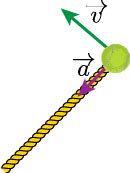A ball is spun around on a string. The tension force is always directed towards the center of the circular path.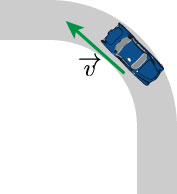A car traveling around a curve is acted on by the static friction force.Earth around stays in orbit due to the radially directed force of gravity between the earth and the sun.Let's spin a ball on a string far away from the earth so there is no gravity acting on the ball. Thus the only force is present is the tension from the rope. $$\sum F_r = T$$

Putting this into Newton's second law, we'll have: $$T = m a_\textrm{cent} = m\frac{v^2}{r}$$

A string will break if the tension in it is greater than 500 N. How fast can I swing a 1 kg ball using 2 meters of this string without breaking it? I'm swinging it in a horizontal plane (disregard gravity for now).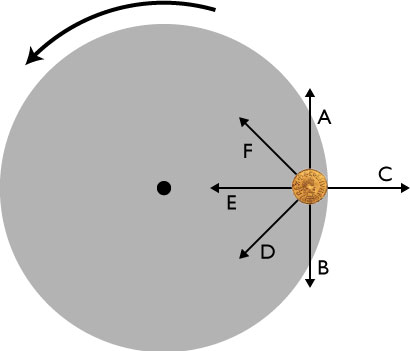A coin sits on a rotating turntable. At the instant shown in the figure, which arrow gives the direction of the frictional force on the coin?

The static friction between the metal coin and the wood will be strong enough to keep the coin from slipping, provided the $v$ of the wooden plate doesn't get too large. Here, the only force acting the radial direction is the $f_s$. Thus:

$$\sum F = f_s = m \frac{v^2}{r}$$

What’s the maximum speed my 200 kg go-kart can go around a curve with radius 10m without sliding out of control? (Assume rubber tires on dry concrete.)

### So far...

Objects traveling around in a circle, even with constant speed, have a centripetal acceleration, given by:

$$a_\textrm{cent} = \frac{v^2}{r}$$

This is a vector, and always pointed towards the center of the circle around which the object is traveling. Also pointing towards the center of the circle is a centripetal force. This could be tension, friction, gravity, etc...

$$\mathbf{F}_\textrm{cent} = m \mathbf{a}_\textrm{cent}$$

The period of an object undergoing uniform circular motion is given by:

$$T = \frac{2 \pi r}{v}$$

### x-y coordinate systemsFor many situations, an x-y coordinate system is a great idea.

### Other coordinates...

For others, not so much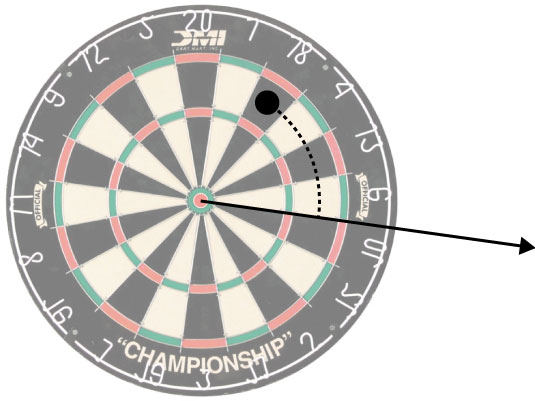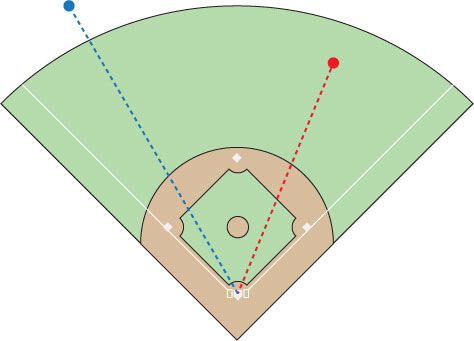If we walk around a circle, we can describe our position with respect to the center by two variables: $\theta$ and $r$.

We could also use $x$ and $y$, but we can see how just using an angle and a radial distance is easier.1. $\frac{\Delta r}{\Delta t}$
2. $\frac{\Delta \theta}{\Delta t}$
3. $\frac{\Delta x}{\Delta t}$
4. $\frac{\Delta y}{\Delta t}$

If a particle is moving in a circle that is situated in the x-y plane at a constant speed, which of the following quantities will be zero?1. $\frac{dr}{dt}$
2. $\frac{d\theta}{dt}$
3. $\frac{dx}{dt}$
4. $\frac{dy}{dt}$

If a particle is moving in a circle that is situated in the x-y plane at a constant speed, which of the following quantities will be zero?

"The length of an arc of a unit circle is numerically equal to the measurement in radians of the angle that it subtends"

### Circle MathIf $\theta$ is measured in radians, the distance around the curved path of the circle can be found by:

$$\theta = \frac{s}{r}$$

This makes sense considering:

$$\theta_\textrm{full circle} = \frac{s}{r} = \frac{2 \pi r}{r} = 2 \pi \rm \;rad$$

### Period

A sometimes more useful measure of the orbit of an object is its period, $T$. The period is defined as the time it takes to complete one revolution around. For example:

1. The period of the moon around the earth: 27.32 days = $2.36 \times 10^6$ s
2. The period of a LP record is 1.8 s (33 $\frac{1}{3}$ rot per minute)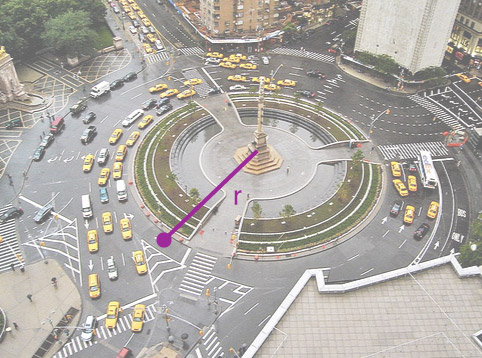The radius of Columbus Circle is 0.025 miles (1/40th of a mile). If you want to drive around it in 5 seconds (1/720 hours). How fast should you be going?

Here is a ball being swung in a vertical circle. Among the points listed, where is the tension in the string the greatest?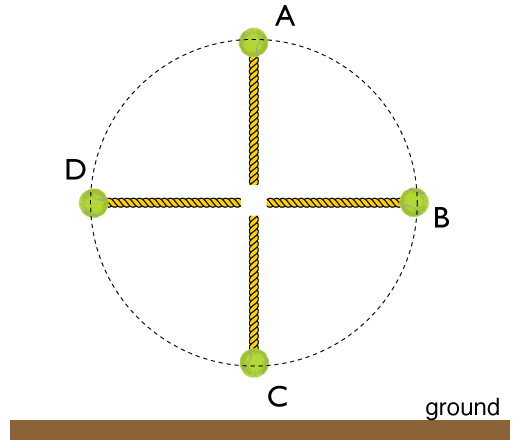What is the normal force acting on the mass $m$ if the tray is being accelerated in the direction of +y with a magnitude of...?### Swinging Scales

At the position shown, what is the minimum speed the box can have while still touching the tray?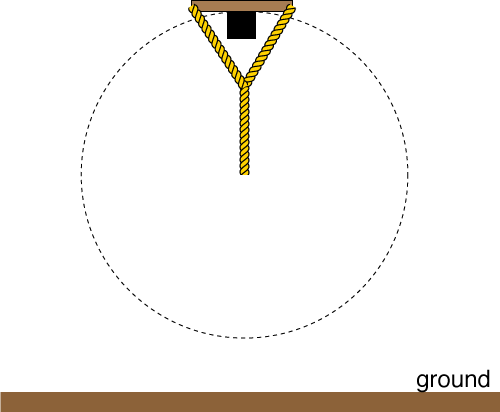A space station is designed in the shape of a large, hollow ring that is uniformly rotating. The outer diameter of the station is 300 m. With what period must the station rotate so that a person sitting on the outer wall experiences “a moon like gravitational feel".

Which of the vectors best shows the net acceleration of the ball at the location shown?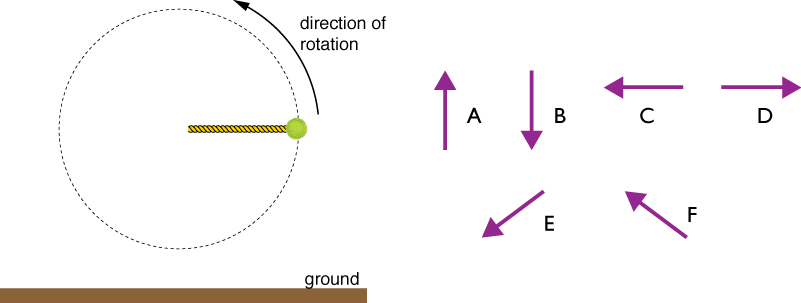At the position labeled $A$, what is the minimum speed the ball can have while still keeping some tension on the rope? (i.e how fast to ensure tension cannot be zero)